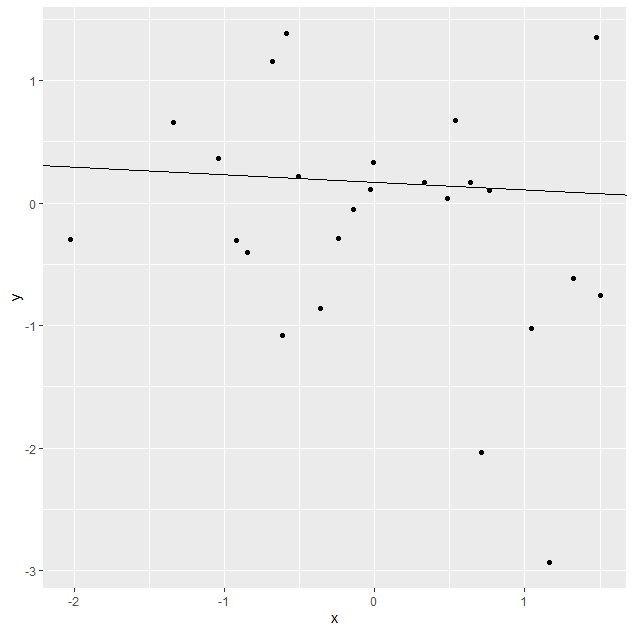# How to change the abline colour created using ggplot2 in R?

To change the abline color created using ggplot2 in R, we can follow the below steps −

• First of all, create a data frame.

• Then, use ggplot2 to create the regression line with given slope and intercept.

• After that, create the same plot with colour argument to change the abline colour.

## Example

#### Create the data frame

Let’s create a data frame as shown below −

x<-rnorm(25)
y<-rnorm(25)
df<-data.frame(x,y)
df

## Output

On executing, the above script generates the below output(this output will vary on your system due to randomization) −

        x            y
1   -0.51902492 -0.79354642
2   -0.42864943 -0.25896843
3    0.57382031  0.95552909
4   -1.68136435  0.19044111
5   -0.69830456 -0.01767888
6   -0.76028497 -0.19016998
7   -0.16044373  0.30392163
8   -1.30390581  0.00911881
9   -0.92685295 -0.63172007
10   2.05518766 -0.70310776
11   0.40343320  1.14764580
12  -1.01411102  0.29829312
13  -0.39075157  0.06896220
14   1.71747493  0.18522334
15   0.73520133 -0.01642746
16   0.19556420  0.55860325
17  -1.45367281  0.01375643
18  -2.42102756  0.63214669
19   0.16466908 -1.25939472
20  -0.88376161 -1.02222998
21  -0.68787689 -0.85754925
22  -0.33156054  0.38232947
23   0.14147640 -0.04030800
24   0.39239316 -0.46167568
25  -0.09390706 -0.27455582

Create the regression line plot

Using ggplot2 to create the regression line with given slope and intercept as shown below −

x<-rnorm(25)
y<-rnorm(25)
df<-data.frame(x,y)
library(ggplot2)
ggplot(df,aes(x,y))+geom_point()+geom_abline(slope=-0.062712, intercept=0.165886)

## OutputCreate the regression line plot with different line colour

Using ggplot2 to create the regression line with given slope and intercept having different line colour as shown below −

x<-rnorm(25)
y<-rnorm(25)
df<-data.frame(x,y)
library(ggplot2)
ggplot(df,aes(x,y))+geom_point()+geom_abline(slope=-0.062712,
intercept=0.165886,colour="green")

## Output